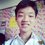# Graph Theory Notes Part 1

Recently, I have started learning graph theory, here are a list of terminologies that are commonly used in graph theory,

• A graph $G=(V,E)$ is a collection $V$ of vertices or nodes and $E\subset V \times V$ of edges.

• Two vertices are adjacent if there is an edge that has them as endpoints. An edge and a vertex are said to be incident if the vertex is an endpoint of the edge.

• If $U$ is a subset of the vertices, then the induced subgraph $G[U]$ is the graph obtained by deleting all vertices outside $U$, keeping only edges with both endpoints in $U$.

• The complement $\overline {G}$ of $G$ is a graph with the same vertex set as $G$ and $E(\overline{G})=\{e \notin E(G)\}$.

• A graph is bipartite if the vertex set can be partitioned into two non-empty disjoint sets $V_1 \cup V_2$ such that edges only run between $V_1$ and $V_2$ or there are no edge that has both endpoints in the set.

• A graph is complete bipartite if $G$ is bipartite and all possible edges between the two sets $V_1, V_2$ are drawn. In the case where $|V_1|=m, |V_2|=n,$ such a graph is denoted by $K_{m,n}$.

• Let $k\geq 2.$ A graph $G$ is said to be $k$-partite if the vertex set can be partitioned into $k$ pairwise disjoint sets $V_1, V_2, \cdots , V_k$ such that no edge has both endpoints in the same set.

• A complete k-partite graph is defined similarly as a complete bipartite. In the case where $|A_i|=n_i$, such a graph is denoted by $K_{n_1,n_2,\cdots ,n_k}$. (Note that a 2-partite graph is simply a bipartite graph.)

• An edge whose endpoints are the same is called a loop.

• A graph where there is more than one edge joining a pair of vertices is called a multigraph.

• A graph without loops and is not a multigraph is said to be simple.

• A graph is planar if it is possible to draw it in the plane without crossing any edges.

• The chromatic number of a graph is the minimum number of colours needed to colour the vertices without having the same colour for any two adjacent vertices.

• A clique or complete graph on $n$ vertices, denoted $K_n$, is the $n$-vertex graph with all $\binom n2$ possible edges.

• A path is a sequence of distinct, pairwise-adjacent vertices. (A graph itself can also be called a path.) The length of a path is defined to be the number of edges in the path.

• A walk is a sequence of not-necessarily-distinct, pairwise-adjacent vertices.

• A cycle is a path for which the first and last vertices are adjacent. (A graph itself can also be called a cycle.) The length of a cycle is defined to be the number of vertices (or edges) in the path.

• The degree $d(v)$ of a vertex $v$ is the number of edges that are incident to $v$.

• The distance between two vertices $u,v$ in a graph is defined to be the length of the shortest path joining $u,v$. (In the case the graph is disconnected, this may not be well-defined.)

• A graph is connected if for any pair of vertices, there exists a path joining the two vertices. Otherwise, a graph is disconnected.

• A tree is a connected graph with no cycles.

• A forest is a not-necessarily-connected graph with no cycles.Note by ChengYiin Ong
8 months, 4 weeks ago

This discussion board is a place to discuss our Daily Challenges and the math and science related to those challenges. Explanations are more than just a solution — they should explain the steps and thinking strategies that you used to obtain the solution. Comments should further the discussion of math and science.

When posting on Brilliant:

• Use the emojis to react to an explanation, whether you're congratulating a job well done , or just really confused .
• Ask specific questions about the challenge or the steps in somebody's explanation. Well-posed questions can add a lot to the discussion, but posting "I don't understand!" doesn't help anyone.
• Try to contribute something new to the discussion, whether it is an extension, generalization or other idea related to the challenge.
• Stay on topic — we're all here to learn more about math and science, not to hear about your favorite get-rich-quick scheme or current world events.

MarkdownAppears as
*italics* or _italics_ italics
**bold** or __bold__ bold
- bulleted- list
• bulleted
• list
1. numbered2. list
1. numbered
2. list
Note: you must add a full line of space before and after lists for them to show up correctly
paragraph 1paragraph 2

paragraph 1

paragraph 2

[example link](https://brilliant.org)example link
> This is a quote
This is a quote
    # I indented these lines
# 4 spaces, and now they show
# up as a code block.

print "hello world"
# I indented these lines
# 4 spaces, and now they show
# up as a code block.

print "hello world"
MathAppears as
Remember to wrap math in $$ ... $$ or $ ... $ to ensure proper formatting.
2 \times 3 $2 \times 3$
2^{34} $2^{34}$
a_{i-1} $a_{i-1}$
\frac{2}{3} $\frac{2}{3}$
\sqrt{2} $\sqrt{2}$
\sum_{i=1}^3 $\sum_{i=1}^3$
\sin \theta $\sin \theta$
\boxed{123} $\boxed{123}$

## Comments

There are no comments in this discussion.

×

Problem Loading...

Note Loading...

Set Loading...